# Implementing quantum dot qubits in optimized linear quantum computing architectures through evolutionary computational modeling

0
2470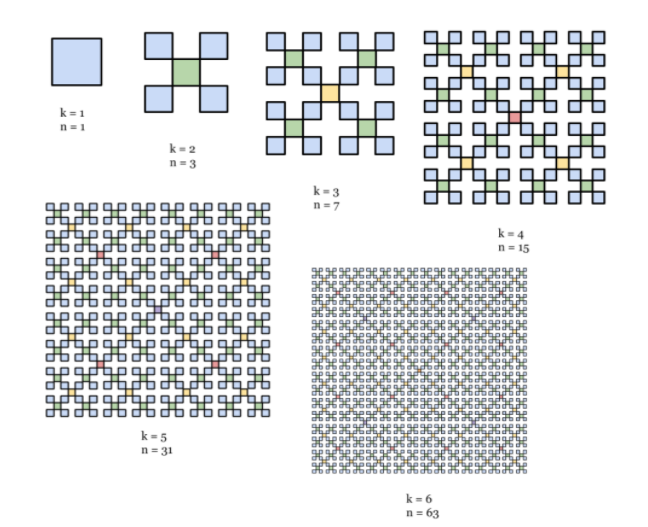Author: Lucas Braun

Peer Reviewer: Hyunjin Lee

Professional Reviewer: Melissa Shell

School of Science and Technology

Segmented linear quantum computing architectures provide high error tolerance while maintaining a simple, one-dimensional layout. However, research on implementing currently-achievable qubits in segmented architectures is limited, and the performance of such architectures in full-scale processors is yet to be evaluated. This research analyzed the usability of current quantum dot, ion trap, and transmon qubits in segmented architectures. The theoretical performance of quantum dot qubits was evaluated in megaqubit-scale processors.

Models based on current research were used to analyze large numbers of segmented designs. Architecture parameters and physical error rates were used to approximate qubit speeds, sizes, and logical error rates. An evolutionary algorithm generated optimally-compact arrays of logical qubits to evaluate full-scale processors. Segment specifications were then incorporated to determine processor speed and size.

Quantum dots produced low-error qubits with fast speeds and small sizes (0.9mm2, 186.7 gates per second). Ion traps achieved low error rates but had large sizes and slow speeds (15.7mm2, 0.184gps). Transmon qubits were fast (59.15gps) but too large to be practical (over 1m2). Analysis of ten qubit array sizes showed that optimal arrays mimic box fractals. Quantum dots in 10-by-10 arrays were estimated to produce 18.30cm2 processors capable of 18429gps.

The results indicate that quantum dots are strong candidates for efficient processors using segmented architectures. The qubit arrays suggest that fractaline structures allow for optimally compact qubit arrangement. These results can guide future research in scalable quantum computing and suggest that megaqubit-scale quantum dot processors are currently viable.

##### I. INTRODUCTION

A. Background

In recent years, quantum computing has developed as a way to represent data in the form of superpositions of possible binary quantum states (qubits), most often up and down quantum spins [1-6]. In theory, this allows for repetitive tasks to be performed very rapidly. A number of repetitive tasks performed by quantum computers, such as Shor’s algorithm and boson sampling, have become central to illustrating the supremacy of quantum devices over classical devices [1,7,8]. However, the implementation of effective quantum devices has proven difficult, as error rates are frequently very high and error correction very costly [1-4]. Thus, a main focus of developments in quantum computing has been to minimize error rates and to develop efficient layouts for qubits and their classical electronic overhead (together called qubit architectures).

1.Current Architectural Designs

A variety of architectures for quantum computing have been proposed, with heavy emphasis on two-dimensional (2D) arrays of qubits which are able to be quantum entangled (coupled) with nearest-neighbor qubits . However, 2D qubit arrays are often not feasible on a large scale with today’s classical computational technology due to the need for the classical electronics which manipulate qubits to access each qubit [2, 3]. Thus, we may turn to one-dimensional (1D) quantum computing architectures, which are currently viable [1, 9].

In 1D designs, qubits are arranged in lines, and all classical mechanisms needed to operate the quantum computer (classical control mechanisms) are kept in the same plane as the qubits. This allows for simpler control of qubits and is not feasible for 2D qubit arrays [1,2,9].

However, 1D qubit arrays do have significant drawbacks compared to 2D arrays. Because each qubit is neighbored by only two qubits instead of four, most 1D designs only let each qubit connect to two qubits. This means that there is less interconnectivity among qubits, so more complex algorithms are required to stabilize quantum states for use in computing, leading to more opportunities for errors. 1D architectures also need more qubits to be able to implement algorithms such as those for error correction, leading to less than efficient use of qubits. Thus, 1D designs tend to be slower and less space efficient than 2D designs.

To provide a compromise between standard 2D and 1D designs, 1D architectures can implement segmented chains of qubits. In segmented chains, groups of qubits called segments are all able to be coupled with each other. These segments are in turn linked by a single ‘shuttle qubit’ which couples with all of the data qubits in each segment, as well as neighboring shuttle qubits . Segmented designs provide the advantage of greater connectivity among qubits over traditional nearest-neighbor-only 1D designs. This in turn provides opportunities for flexible architecture arrangements and error correction methods [1, 9]. The main drawback of segmented designs is that, compared to other 1D layouts, segmented 1D architectures may have lower error thresholds for algorithm implementation . This being said, the drawbacks of 1D segmented qubit arrays are able to be addressed using current technologies. This makes 1D segmented arrays prime targets for near-term quantum computing, as well as potential targets for low-error quantum computing in the future [1, 2].

2. Error Correction in 1D Segmented Chains

In order to be able to correct errors in quantum computations, error correction routines called surface codes are commonly implemented (in both 1D and 2D architectures) to couple and measure different qubits, thereby stabilizing the system [1, 2]. For a 1D segmented chain with d qubits per segment (not including the shuttle qubits), 5(2d-1) rounds of coupling are needed per round of stabilizing . The surface code also groups 2d-1 segments into a single logical qubit. There is one shuttle qubit per segment (since each shuttle qubit is shared by the segments which use it) Thus, there are d+1 total physical qubits per segment and 2d+1 segments per logical qubit, meaning (d+1)(2d-1) physical qubits are needed per logical qubit, including shuttle qubits. Additionally, in order for logic gates to be performed while repeatedly stabilizing with surface code, it is often necessary to use multiple qubit segments per gate. The simplest way to fix this requires that not all segments are used to store data; it has been shown that using every fourth segment to store data allows logic gates to be fault-tolerant .

The stabilizing process is performed repeatedly during each computation. Thus, the possibility that physical errors will occur during rounds of stabilizing must be taken into account. Correcting errors caused by the surface code can be done by implementing a gauge code which uses multiple logical qubits to form a single logical qubit with a reduced error rate (called the logical error rate). These logical qubits can in turn be grouped to form qubits which, for low physical error rates, have further decreased logical error rates, thereby increasing computational accuracy at the expense of efficient qubit use [1, 9]. The level of concatenation of the gauge code (n) is defined as the number of times lower-level qubits are combined to form a higher-level qubit. Six lower-level qubits are used per higher-level qubit, so the number of segments per logical qubit is given by: N= 4 x 6^n. The lowest two levels of concatenation are unable to properly fix errors; thus, we need n>3. After the gauge and surface codes are applied, a total of 4 x 6^n(d+1)(2d-1) physical qubits per logical qubit are needed .

To utilize the gauge code, we must find the rate of errors which persist after the surface code is applied and again after the gauge code is applied. This error rate must be calculated and is the quantum processor’s true logical error rate. Li and Benjamin  provide an effective model for doing so, based on the assumption that errors only occur when initializing a quantum state, when measuring a quantum state, or when performing logic gates using one or two qubits. They approximate the error rates for initializing, measuring, and performing two-qubit gatesas being roughly equal and roughly ten times the error rate of single-qubit gates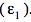. Li and Benjamin’s model (and the models used in this paper) do not take into account error due to imperfections in the experimental setup, as they vary greatly between setups and are difficult to account for in models . Using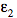, we can find the error rate for a single round of stabilizing. It has been found that, for any type of 1D array setup, whenis below a certain threshold, the error rate per round decreases as the number of qubits per segment increases. Otherwise, the error rate increases with number of qubits per segment. This threshold has been found to be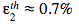. Assuming thatis independent of d, the error rate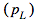for a single round of stabilizing is accurately modelled by:for segmented 1D architectures, where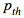= 0.02 is the error rate per round of stabilizing achieved when. Note that this formula is highly accurate for low numbers of qubits per segment but tends to significantly over-report error rates for more than ten qubits per segment.

Similar to producing fault-tolerant surface code setups, establishing a fault tolerant method for performing logic gates using a 1D segmented array requires the scaling-up of existing processes. To implement a CNOT gate (a basic logical unit of quantum processing) with a sufficiently low error rate, we require a process that involves a total of 14 wave function initializations, wave function measurements, and two-qubit gates . While performing each of these processes, roughly d rounds of stabilizing are needed. Thus, the error rate for one CNOT gate is given by:

We can use the error rate per CNOT gate to determine whether or not we need to use a gauge code and, if so, what level of concatenation is needed . Li and Benjamin  found that a threshold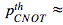4×10^-6 exists below which the error rate per CNOT gate decreases greatly with concatenation level. Thus, in order to be able to implement the gauge code, the error rate per CNOT gate must be below this level. The error rate per CNOT gate at a given level of concatenation is given by: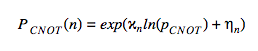where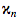and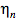are arbitrary curve-fitting parameters which depend on n. Li and Benjamin  found through computational modeling that the values for these parameters for the first four levels of concatenation are:

 n1 0.9973 4.1141 2 1.0303 5.8552 3 2.0717 18.7274 4 3.4795 36.4548

The error rate per CNOT gate must be below a certain threshold to perform any given computation reliably. This paper uses 10^-18, as this is approximately the upper bound on error in current classical CPUs . The specific error rate needed depends on the algorithm being implemented; note that the average number of CNOT gates performed between logical errors is given by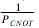[1, 9].

3. Physical Qubit Types

Thus far, ion trap qubits and superconducting transmon qubits have been tested in segmented 1D architectures , though other qubit types have been proposed. Some types of qubits, such as electron spin quantum dot qubits, have been implemented previously in 1D architectures but have not been used in all-to-all connected 1D segments, even though all-to-all connection has been shown to be attainable [11-13].

Ion trap methods use long, narrow potential wells to trap ions in a line . These wells are placed end-to-end, and the qubits at the end of each well act as shuttle qubits. In resonators using superconducting transmon qubits, resonators are similarly placed end-to-end and share one qubit as the shuttle qubit [1, 15]. When using quantum dot qubits, the specific mechanism used to store data may vary, but one of the most common mechanisms is to use the spin of a single electron or a single electron hole trapped in a quantum dot in silicon or germanium [4, 5, 13, 16]. The type of qubit used can greatly alter the physical properties of the architecture. Most notably, ion trap qubits are not solid state, whereas superconducting transmon qubits are solid state .

Physically-achievable error rates and optimal gate times vary with qubit types, as will methods for constructing the qubit array. For instance, superconducting transmon qubits have been found to have optimal two-qubit error rates of about=0.56% and single-qubit error rates of about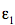=0.06% . Transmon qubits also have optimal two-qubit gate times on the order of 50-200 ns . Ion traps have been shown to have even lower two-qubit error rates, at about 0.1% . However, ion trap qubits have much slower optimal two-qubit gate times, on the order of 90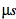.

Quantum dots are strong candidates for physical qubits in 1D segmented chains, but have not yet been implemented in practice. It has been shown that silicon-based quantum dots have some of the lowest physical error rates, as low as 0.003% in electron spin quantum dots . These qubits have two-qubit gate times on the order of 500 ns. Germanium-based quantum dots are also strong candidates, due to their strong energy level splitting and hole mobility . However, the logical error rates of Ge quantum dots have not been thoroughly evaluated, making it difficult to evaluate their theoretical feasibility in 1D segmented arrays . Both germanium- and silicon-based quantum dots have the additional advantage of being able to be fabricated using similar technologies to those used to create classical computer chips [4, 5, 13, 16]. They are also multiple orders of magnitude smaller in area than other qubit types [12, 18]. It is thus worth investigating possible implementations of quantum dot qubits in 1D segmented architectures.

B. Purpose

This research developed models which expanded upon previous models to evaluate the error rates and efficiencies of a variety of possible hardware choices and physical conditions for 1D architectures. This allowed for scaled-up processors with optimal speeds, sizes, and error rates to be designed. Emphasis was placed on evaluating Si quantum dot qubits due to their small size, quick physical gates, and low physical error rates. Ion trap and superconducting transmon qubits were also evaluated for comparison. The parameters of physical error rate, logical error rate, qubit use efficiency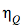(one over the number of physical qubits per logical qubit), and gate use efficiency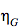(one over the number of physical gates performed per logical gate) were used to determine the usability of different architectures in large-scale arrays . This allowed for the design of theoretically optimized 1D segmented architectures for each set of specifications. Specific gate times, selected based on current optimal gate times for different hardware, were also considered to determine processor speed .

This research also explored new arrangements of 1D segmented chains in the form of segments which are able to couple with multiple other segments in a branching structure. Such arrangements should be possible to manufacture using similar qubit setups to those used in 2D arrays for junctions. If done in such a way that one or both sides of each segment are accessible by classical control mechanisms in the same plane as the segments, this could help make quantum processors more compact while still allowing for the simpler computational overhead of 1D arrays [1, 2]. The one main complication which this causes is managing the overhead for node qubits with less lateral access than for other qubits. However, such difficulties should be able to be overcome through a minor rearrangement of the classical computational overhead [4, 5, 13, 16].

To evaluate the possibility of branching segmented arrays, this research used a theoretical computer design in which segmented chains containing d physical qubits were arranged in a perpendicular lattice that held n logical qubits in each direction (total size n x n logical qubits). An evolutionary algorithm was implemented to automatically optimize the array’s compactness.

The models used in this research therefore evaluated the feasibility of different qubit types in scalable 1D architectures. The models also designed theoretically optimal 1D qubit chains and chain arrangements. The development of such optimal architectures provides a step towards the development of scalable quantum processors for individual quantum computers and large-scale quantum networks. .

##### II.RESULTS

The following table summarizes the physical error rates of the qubit types used in this research:

 Qubit Type Approximate Physical Error Rate (as implemented in this research’s models) Ion Traps 6.20 x 10^-4 Superconducting Transmons 3.82 x 10^-3 Electron Spin Quantum Dots 3.00 x 10^-5Figure 1: Logical error rate versus efficiency for gate use efficiencies and qubit use efficiencies and for a range of possible two-qubit physical error ratesData points shown as circles represent logical qubits with gauge code concatenation of n=3. Points shown as triangles are logical qubits with n=4. For the qubit use efficiency graph, data with qubit use efficiencies below 10^-6 are excluded to illustrate a likely threshold for the size of quantum processors. For both graphs, the horizontal dashed line corresponds to the logical error rate target of 10^-18. For the gate (qubit) use efficiency graph, the vertical line corresponds to the gate (qubit) use efficiency target of 9 x 10^-5 (4.59 x 10^-6).

A. Optimal Logical Error Rate for Given Physical Error Rate and Efficiencies

Logical error rates were computed as functions of physical error rate and either gate use efficiency or qubit use efficiency, as shown in figure 1.

When considering gate use efficiency (and the approximate logical gate times which can be derived from it), a target gate use efficiency was arbitrarily chosen to be about 9 x 10^-5, corresponding to 10-100 gates per second (gps) for most qubit types. At this speed, quantum dot qubits showed logical error rates of about 10^-24 and speeds of about 200 gps, using gauge code level n=4. This error rate is highly comparable to the error rates of classical CPUs . Logical qubits implementing ion trap qubits with the same gate use efficiency would have logical error rates of approximately 8.30 x 10^-2. For logical qubits using superconducting transmons, error rates below unity could not be achieved for>10-6.

When considering qubit use efficiency (which is directly correlated to processor size), a target qubit use efficiency of 4.59 x 10^-6 was chosen. We can see in the second graph in figure 1 that, for all logical qubits which meet this target, using a gauge code concatenation level of n=3 will produce a lower logical error rate than n=4. At this qubit use efficiency, quantum dot qubits showed logical error rates of about 5.14 x 10^-21 and formed logical qubits of sizes of about 0.218 mm2. Ion trap qubits of the same qubit use efficiency showed logical error rates of about 1.16 x 10^-4, which does not meet the threshold of 10^-18 to be comparable to classical error rates. For transmon qubits, the computational model used here predicts logical error rates greater than 1.0. This result does not make physical sense but is an expected result of the model for scenarios with very high logical error rates (the model slightly over-predicts logical error rates). This indicates that implementing transmon qubits would not allow for qubit use efficiencies above 10^-6 while having logical error rates low enough to run algorithms.

The data in figure 1 suggest that noise-resistant quantum dot qubits would be most optimal for implementing scalable 1D segmented chains of qubits. However, this assumes that two-qubit gates can be realized on non-neighboring qubits with fidelities comparable to nearest-neighbor fidelities . A segmented quantum dot processor would have a sufficiently low error rate to implement common algorithms which use quantum computers, such as Shor’s algorithm, and have error rates comparable to those of classical processors . Since it is impractical to optimize for both gate use efficiency and qubit use efficiency simultaneously, one could either develop a processor which is optimized for gate use efficiency (speed) or qubit use efficiency (size). Given current quantum dot sizes (on the order of 500-1000 nm, including classical control [4, 5, 9, 13]), the processor would have logical qubits of area approximately 0.220 to 0.881 mm2 (qubit use efficiency of 1.13 x 10^-6), not including classical control, if optimized for speed. If optimized for size, the processor would have logical qubits of area approximately 0.0544 to 0.222 mm2 (qubit use efficiency of 4.59 x 10^-6). The processor would be able to run at speeds on the order of 187 two-qubit gates per logical qubit per second (gate use efficiency of 9.34 x 10^-5) if optimized for speed, or 124 two-qubit gates per logical qubit per second (gate use efficiency of 6.18 x 10^-5) if optimized for size.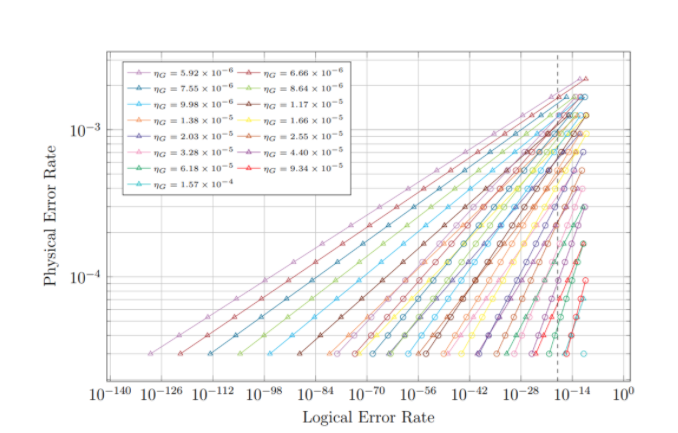Figure 2: Physical error rate as a function of logical error rate and gate use efficiencyData points shown as circles represent logical qubits with gauge code concatenation of n=3. Points shown as triangles are logical qubits with n=4. Points with logical error rates above 10^-10 are excluded to provide a better picture of the physical error rate ranges which are likely to be useful when constructing quantum processors. The vertical dashed line corresponds to the logical error rate target of 10-^18.

B. Optimal Physical Error Rate for Given Logical Error Rate and Efficiencies

The impact on physical error rate of changing logical error rate, qubit use efficiency, and gate use efficiency was evaluated to find the maximum physical error rate for which an accurate quantum processor could be developed under different spatial and temporal constraints. Graphs of the generated data are shown in figures 2 and 3.

The data in figure 2 show that logical error rates below 10^-18 can be achieved within the given parameter ranges for physical error rates as high as 1.67 x 10^-3; this physical error rate is currently achievable in most quantum dot qubits and is close to being achievable in ion trap qubits. However, this versatility comes at the cost of low gate and qubit use efficiencies: 5.92 x 10^-6 and 7.77 x 10^-8, respectively, which correspond to processing speeds of approximately 11.83 two-qubit gates per logical qubit per second and logical qubit sizes of approximately 12.9 mm2.The data were used to determine a theoretical cutoff for maximum usable physical error rate for a minimum processor speed. Let us take the minimum allowed gate use efficiency for a given processor to be 10^-5 (corresponding to 20 gps for 500 ns gates). With such a constraint, the maximum physical error rate required to obtain logical error rates comparable to classical error rates is approximately 5.29 x 10^-4 for n=3 or 9.39 x 10^-4 for n=4. These both would allow logical error rates on the order of 10^-21 to be achieved. The processor would have a qubit use efficiency of approximately 9.08 x 10^-7 for n=3 or 1.51 x 10^-7 for n=4. These correspond to logical qubit sizes of about 0.110 mm2 for n=3 or 0.660 mm2 for n=4 (if implementing quantum dots).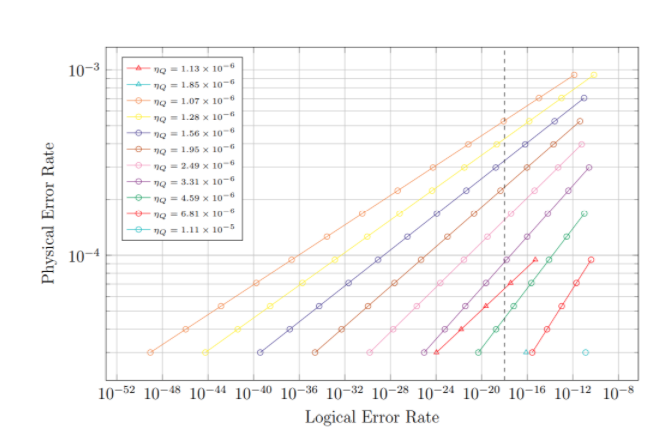Figure 3: Physical error rate as a function of logical error rate and qubit use efficiencyData points shown as circles represent logical qubits with gauge code concatenation of n=3. Points shown as triangles are logical qubits with n=4. Points with logical error rates above 10^-10 are excluded to provide a better picture of the physical error rate ranges which are likely to be useful when constructing quantum processors. Points with qubit use efficiencies below 10^-6 (corresponding to logical qubit sizes on the order of 1 mm2) were excluded to contrast logical qubits with n=3 and those with n=4. The vertical dashed line corresponds to the logical error rate target of 10^-18.

The data in figure 3 show that the maximum physical error rate needed to obtain logical qubits with logical error rates below 10^-18 while having a qubit use efficiency of at least 10^-6 is 3.97 x 10^-4. This physical error rate will produce logical error rates of 2.12 x 10^-19 for qubit use efficiencies of 1.28 x 10^-6 and n=3. This setup would be reasonably sized (about 0.779 mm2 per logical qubit if quantum dot qubits are used) and would run at speeds on the order of 33.2 gps for 500 ns gates. Two-qubit physical error rates of 3.97 x 10^-4 or lower have been demonstrated in silicon quantum dot qubits, and current ion trap two-qubit error rates are approaching this threshold [6, 14, 17].

The data also provide a means of finding the theoretical maximum physical error rate allowed for a certain minimum qubit use efficiency. Taking the minimum qubit use efficiency to be 2 x 10^-6 and implementing quantum dot qubits, logical qubits would have areas on the order of 0.5 mm2. This requirement would indicate a maximum physical error rate of 1.26 x 10^-4 for n=3 to achieve logical error rates below 10^-18. This physical error rate is currently attainable with noise-optimized quantum dots, though it is still several times lower than the lowest transmon qubit or ion trap qubit physical error rates [14, 15]. If using quantum dot qubits, this processor would have a gate use efficiency of 3.28 x 10^-5 and a qubit use efficiency of 2.49 x 10^-6. These values correspond to speeds of approximately 65.7 two-qubit gates per logical qubit per second and logical qubit areas of about 0.401 mm2. Similar logical error rates would not be achievable with n=4 for current physical error rates and qubit use efficiencies above 10^-6.

Thus, ion trap and quantum dot qubits are successful at meeting reasonable physical error rate thresholds while transmon qubits are not. Quantum dot qubits are the smallest and fastest, producing over 60 gps using logical qubits smaller than 1 mm2.

C. Optimal Efficiencies for Given Physical and Logical Error Rates

Gate and qubit use efficiencies treated as functions of logical error rate are shown in figure 4. This way of visualizing the qubit chain data allows for the optimal achievable gate and qubit use efficiencies for given logical error rates to be determined.

The data shown in the first graph in figure 4 allow us to find the highest possible gate use efficiency which can currently be obtained while also achieving logical error rates below 10^-18. These data indicate that the optimal gate use efficiency is approximately 9.34 x 10^-5, resulting in a logical error rate of about 1.08 x 10^-24 if implementing electron spin quantum dot qubits. These logical qubits would run at about 186 gps. Using quantum dot qubits, the optimal gate use efficiency is approximately 1.66 x 10^-5, producing a logical error rate of about 1.69 x 10^-22 and logical gate speeds of about 0.184 gps (one gate every 1/0.184 = 5.4 seconds). The data show that logical error rates below 10^-18 are not achievable with the given parameter sets for transmon qubits, meaning that no optimal gate use efficiency can be found for transmons.

We can also deduce the lowest logical error rate which can be obtained for a processor requiring given minimum gate use efficiency (minimum processing speed). Using 10^-5 as the minimum gate use efficiency, the model predicts that this would produce a logical error rate on the order of about 3.45 x 10^-97. This low of a logical error rate is not likely to be achievable or measurable in practice. However, this result does indicate that arbitrarily low logical error rates can be readily achieved within reasonable minimum gate use efficiency restrictions.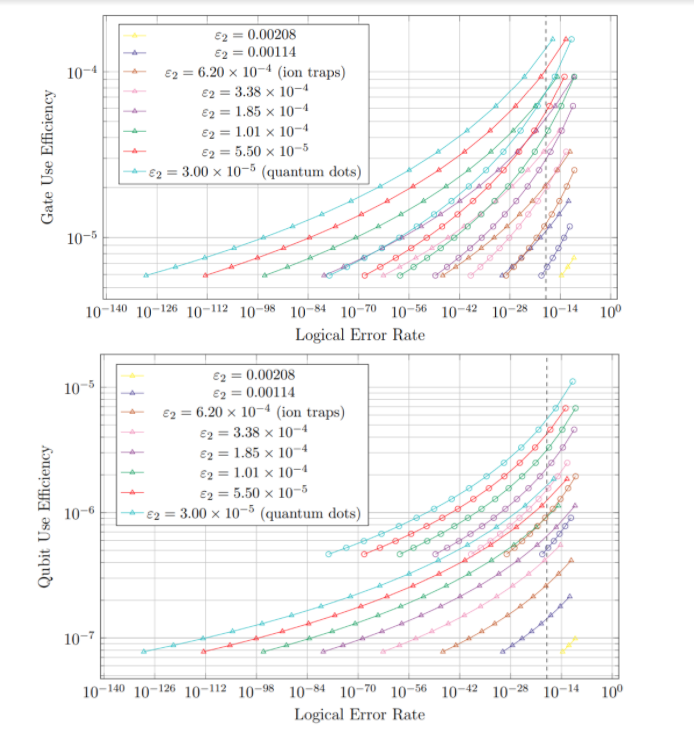Figure 4: Gate and qubit use efficiencies as a function of logical error rate for a range of possible two-qubit physical error ratesData points shown as circles represent logical qubits with gauge code concatenation of n=3. Points shown as triangles are logical qubits with n=4. For both graphs, the vertical dashed line corresponds to the logical error rate target of 10^-18. Unlike for the data in figure 1, the data shown here are cut off at a maximum logical error rate instead of a minimum qubit use efficiency. This allows for a better visual representation of achievable qubit and gate use efficiencies in desirable logical error rate ranges.

The data in the second graph in figure 4 can be used to indicate the highest achievable qubit use efficiency for logical qubits which demonstrate near-classical-CPU logical error rates (below 10^-18). For noise-optimized electron spin quantum dot qubits, the optimal qubit use efficiency is 4.59 x 10^-6, producing a logical error rate of about 5.14 x 10^-21. Ion trap qubits can be implemented with an optimal qubit use efficiency of approximately 7.80 x 10^-7 to produce logical error rates of about 2.68 x 10^-21. As mentioned previously, transmon qubits currently have physical error rates too high to be able to achieve logical error rates below 10^-18. Thus no optimal qubit use efficiency could be calculated for transmons.

We can use the data in the second graph in figure 4 to gauge the lowest achievable logical error rate for a processor which requires a certain minimum qubit use efficiency. Taking 2 x 10^-6 to be the minimum qubit use efficiency which can be used for a given quantum processor, the model used predicts logical error rates of approximately 8.71 x 10^-50. While this low of an error rate is not necessarily achievable in practice, it does illustrate that arbitrarily low logical error rates are achievable with reasonably few physical qubits.

Thus, quantum dot qubits are able to produce fast, compact logical qubits with logical error rates below 10^-18. Ion trap qubits are able to produce sufficiently accurate logical qubits, but not without sacrificing speed or size. Transom qubits are not able to achieve low enough logical error rates within reasonable speed or size restrictions.

D. Summary of Key Results from Qubit Design Optimization

• Highest usable physical error rate: 1.67 x 10^-3 (speed: 11.8 gps, size: 12.9 mm2 if using quantum dots).
• Highest usable physical error rate with logical qubits smaller than 1.0 mm2: 3.97 x 10^-4 (for quantum dots; speed: 33.2 gps, size: 0.779 mm2).
• Optimal gate use efficiencies: 9.34 x 10^-5 for quantum dots, 1.66 x 10^-5 for ion traps, none for transmons (logical error rates below 10^-18 unattainable)
• Optimal qubit use efficiencies: 4.59 x 10^-6 for quantum dots, 7.80 x 10^-7 for ion traps, none for transmons (logical error rates below 10^-18 unattainable)

E. Array Layouts for Different Array Sizes

A probabilistic evolutionary model was used to design optimally compact arrays of logical qubits on a square lattice. An overview of the evolutionary model is provided in section 2.C of the Supplementary Materials. Optimized qubit layouts were generated for n=2 through n=10, as shown in figure 5. (While arrays with n=1 can also be generated, they are a trivial case and are thus not shown, as all segments will always be accessible by classical control. For n=1, the array can optimally hold 4 logical qubits.) Each line segment represents one logical qubit.

The space use efficiency (in units of logical qubits divided by the square of the physical length of each logical qubit) is given by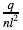where q is the number of logical qubits in the array and l is the physical length of each logical qubit. We arbitrarily let l =1 to ease calculations. The space use efficiency is an indication of the relative compactness of each array layout. Space use efficiency values are shown in figure 6.

It should be noted that, in many cases, the arrays generated here do not perfectly maximize the number of logical qubits per unit area but merely provide a computational approximation. An analytical approximation can be gleaned by noting that “boxes” of four logical qubits arranged in a square can be tiled to create box fractals, as shown in figure 7. This process produces arrays of size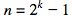and space use efficiencies of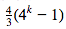, where k is the number of iterations of box fractal generation, starting at 1. Thus, the efficiency of each box fractal array is given by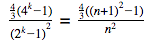. These efficiency values approach 4/3 for large values of n, setting a minimum on the optimal space use efficiency for scaled-up arrays. The analytical and computational solutions are compared in figure 6. The fact that a fractaline structure emerges from the analytical solution suggests that other such fractaline structures could be useful for implementing scalable 1D qubit arrays which do not necessarily conform to a square lattice. (This research uses a square lattice to ensure that sufficient space is allocated for classical control and to simplify computing. However, this is not necessary.)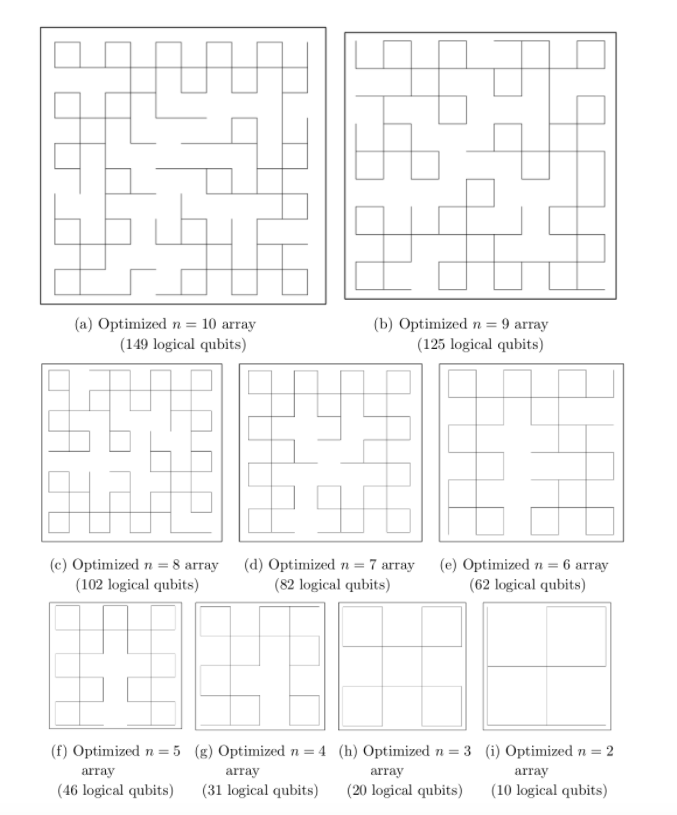Figure 5: Layouts for qubit arrays of different sizesArrays were produced through an evolutionary computational model. Each equal-length segment is one logical qubit.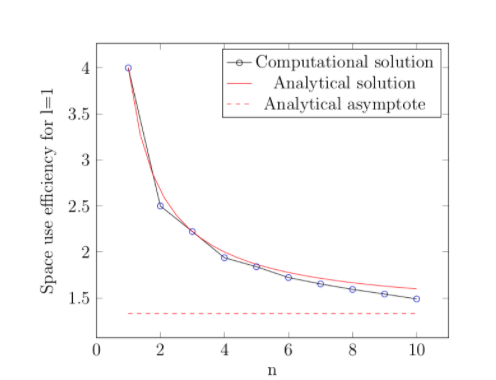Figure 6: Space use efficiency values for arbitrary logical qubits of physical length 1The computational solution data were generated using an evolutionary algorithm, while the analytical solution data used an approximation based on box fractals with space use efficiencies given by ..

F. Implementation of Different Qubit Types in Optimized Arrays

Optimal logical quantum dot qubits were implemented in scaled-up fractaline arrays to analyze how compact and fast the resulting processors could be.

Each logical qubit was organized to minimize the array’s physical size while still allowing classical control access. The amount of space which each physical qubit’s classical control mechanism needs may vary with physical qubit type. To provide variable classical control space, we can arrange the physical qubits in the shape of a square wave of some wavelength. By increasing the wavelength, the amount of access space given to each physical qubit is increased, as shown in figure 8. This system also forces the total amount of classical control space within each array to increase as the amount of access space increases. Note that simply having all physical qubits in each logical qubit be placed in a straight line (corresponding to the maximum possible amount of space provided for classical control) is not likely to be feasible as it would make qubit arrays too large. Such a system would mean, for instance, that qubit arrays implementing noise-optimized quantum dot qubits and having optimally small logical qubits (= 4.59 x 10^-6) would still produce 10 x 10 qubit arrays with areas as high as 4.74 m2.Figure 7: Examples of box fractalsThese fractals provide an analytical solution for optimal space efficiencies of all qubit arrays such that , where k is an integer.

It is difficult to predict what the arrangement of each logical qubit will be due to the variability of each qubit type and classical control mechanism. However, we can make some estimates based on previously proposed designs. For instance, current research suggests that implementing electron spin quantum dot qubits on square waves with wavelengths of 20 physical qubits is viable for quantum dot qubits (wavelengths of about 4 to 20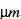) [4, 5, 9, 13]. If we were to implement electron spin quantum dot qubits with d=3 in logical qubits containing 217727 quantum dot physical qubits each (= 4.59 x 10^-6), we could use this wavelength and a large amplitude (500 in this example) to produce optimally compact logical qubits with logical error rates below 10^-18. These logical qubits would be about 4.28 mm long and 1.000 mm wide, including the space provided for classical control.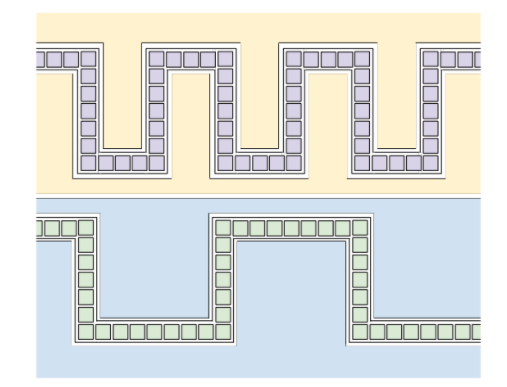Figure 8: Arrangements of physical qubits within a logical qubitEach green or purple square represents one physical qubit. The yellow and blue regions show the space available for classical control immediately around each qubit chain. In the upper arrangement, the square wave of physical qubits has a shorter wavelength. This means that less space is available immediately around each physical qubit but also means that the arrays implementing the arrangement can be more compact. The optimal arrangement may vary significantly with physical qubit type.

If the qubits were implemented in a 10 x 10 array, the array would be about 18.3 cm2 in area and have a processing speed of approximately 18400 gps. Using a box fractal-based design with n=63, as shown in figure 7, the array would be about 726 cm2 in area (26.9 cm on each side) and process at approximately 6.75 x 10^5 gps.

Thus, the results predict that quantum dot qubits can be used in 1D segmented chains to produce compact, low-error quantum computing modules producing about 6.75 x 10^5 gps.

##### III. ERROR AND UNCERTAINTY ANALYSIS

Though there was no experimental error in the data, since the data were generated computationally, the simplifications made by the model and the small number of sample points tested may have caused some error.

The first significant source of error was introduced by the fact that the model used to predict logical error rates became less accurate as d increased. Given that large numbers of physical qubits per segment were generally required for low logical error rate, logical error rate values were generally only accurate to the nearest order of magnitude. However, the model consistently over-predicted the logical error rate of systems, meaning that 1D segmented chain processors should perform better in practice than in the model . Thus, minimum performance thresholds which were met by this model should also be met in practice. Additionally, though the model over-predicted logical error rate, the dependence of the model’s results on the input parameters remained accurate. Thus, though the numerical results of the model are only accurate to one order of magnitude, the comparative performances of different qubit types (with quantum dots performing by far the best) are accurate.

The physical error rates used to predict logical qubit specifications were selected to be representative of the ranges of physical error rates currently achievable. However, their specific numerical values were occasionally only consistent with the physical error rate of a currently-realized qubit type to within 25%. This margin is small compared to the variability of physical error rates (across several orders of magnitude). This inaccuracy was due to the sample size of physical qubits tested in the model and means that there was some slight error in the approximations of the performances of each qubit type. This slightly affects the final numerical results but does not alter the relative qubit performances: the variation between qubit types was far larger than the error in approximating each physical error rate, meaning that comparative physical error rates were preserved. Though the model is capable of computing larger numbers of samples, such computations were not necessary. This is because the physical error rates of specific physical qubits vary sufficiently with physical setup and qubit type that approximations of physical error rate accurate to within half an order of magnitude are sufficient to gauge the relative viability of different qubit types. Using smaller sample sizes when using large ranges also aided analysis by making data easier to process and display. The number of samples needed to eliminate error while still using large parameter ranges would have required more computing power than was reasonable.

During the process of generating qubit arrays using an evolutionary algorithm, a small amount of error was introduced for large qubit arrays. The evolutionary algorithm would at times reach a point where no improvements had been made in the evolutionary gene pool for 23% of the total number of generations and yet the arrays were not fully optimized. This was largely due to the comparatively small populations which were needed for simulations of large arrays to run on a desktop computer in reasonable time. However, the deviation from optimal appears to have been small for lattices with sizes below n=10, as the computational solutions stayed well above the analytical asymptote and continued to level out as expected analytically.

Lastly, variability within each physical qubit type introduced some uncertainty into the logical qubit sizes and logical gate times. Each physical qubit can be built to different sizes and run at different physical gate times, which impacts logical qubit sizes and speeds. There was also uncertainty in the amount of classical control space needed per physical qubit for different qubit types. Though the approximations provided in this paper are based on control systems and qubit designs which have been documented thoroughly and have been shown to work, a wide variety of other designs may be used which could alter the size or speed of the processor. Thus, as individual physical qubits are improved over time, the models developed in this paper can be applied to new qubit developments.

As discussed above, the level of error in this research’s data did not prevent the comparison of qubit types’ performances and the development of efficient architectural layouts. Most forms of error can be reduced in future research by limiting the qubit specifications considered or by using a supercomputer. However, within the scope of this research, computational simplicity and wide parameter ranges were necessary to be able to consider several qubit types while operating with limited computing resources.

##### IV. CONCLUSIONS

Data were generated using a computational model which expanded upon the results of previous segmented chain research to consider scalability, qubit type, and architectural arrangement. The data were analyzed to evaluate the performance of theoretical physical qubits with different physical error rates in 1D segmented chains containing different numbers of physical qubits and implementing different levels of gauge code concatenation .

A. Evaluation of Qubit Types

The data suggest that silicon quantum dot qubits currently provide the most promising logical error rates, computing speeds, and architecture sizes for 1D segmented arrays. Logical qubits implementing electron spin quantum dots in silicon should perform as many as 187 two-qubit gates per second while maintaining logical error rates well below 10^-18 and logical qubit sizes below 0.9 mm2. Alternatively, logical qubits with sizes approaching 0.1 mm2 should be attainable while still maintaining logical error rates well below 10^-18 and processing about 124 logical gates per second. Electron spin quantum dots in silicon also show the best ability to produce arbitrarily low logical error rates for logical qubits smaller than 0.5 mm2 or faster than 100 logical gates per second.

Ion trap qubits are able to achieve logical error rates below 10^-18 in scaled-up 1D segmented arrays but are slow and space inefficient compared to quantum dot qubits. For large numbers of ions, the size of each physical qubit is approximately 12.3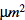, not including classical control (contrasting quantum dot sizes of about 1 m2) [4, 5, 14]. Thus, the smallest that current ion trap logical qubits can be made while achieving logical error rates below 10^-18 is on the order of 15.7 mm2. Individual two-qubit gate times for ion trap qubits are slow: approximately 90 s, compared to 50-200 ns for transmons and about 500 ns for quantum dots [3, 14, 17]. This means that the fastest ion trap qubits which have near-classical-CPU logical error rates would only be able to compute approximately one logical gate every 5.4 seconds. Thus, though ion trap qubits are usable from the standpoint of error rate and scalability, they are likely too slow (and possibly too large) to be practical for solving computational tasks.

Superconducting transmon qubits do not appear to be viable in scaled-up 1D segmented architectures, as current physical error rates in transmon qubits are too high and physical qubits too large for practical implementation. Due to their comparatively high physical error rates (0.56%) and physical sizes (on the order of 0.1 mm2), any 1D segmented logical qubit implementing superconducting transmon qubits would have an area of over one square meter, not including classical control . Such a logical qubit would be several orders of magnitude larger than any logical qubit implementing a different physical qubit type and may thus make it difficult to implement these logical qubits in qubit arrays.

B. Design of Compact Qubit Arrays

An evolutionary algorithm was created and used to generate lattices containing logical qubits. The goal was to fit as many logical qubits as possible into a square lattice of set size while ensuring that at least one side of each logical qubit was accessible to classical control from the outside of the lattice. The resultant arrays of logical qubits (shown in figure 5) provide an approximate solution to this problem and indicate that large numbers of “boxes” arranged in a checkerboard-like pattern near the outside of each array provide optimal qubit packing.

An analytical approximation (shown in figure 7) using box fractals with lattices of sizelogical qubits, where k=1,2,3,…, confirmed this trend. The analytical solution strongly agrees with the computational solution, including for non-integer values of k, as shown in figure 6. The analytical approximation provides a lower bound on the average number of logical qubits per lattice “tile” (space use efficiency) which is approached for large arrays. This bound is 4/3 logical qubits per tile, which shows that using fractaline packing arrangements remains more efficient than merely arranging logical qubits in a line for large arrays. (The space use efficiency limit for the latter scenario is 1.) Overall, the fact that box fractal structures provide useful lattices for arranging of qubits in a quantum processor suggests that similar fractals could efficiently be used in the arrangement of qubits which do not necessarily follow a square grid pattern.

C. Implementation of Quantum Dot Qubits in Fractaline Lattices

Optimal Si quantum dot qubits were implemented in scaled-up arrays to further test their performance. When constructing logical qubit arrays, it was inefficient to have all of the physical qubits within each logical qubit arranged in a straight line. Arranging the physical qubits in a square wave with short wavelength and large amplitude still allows the physical qubits to be accessed by classical control mechanisms while making the logical qubit arrays more compact. Such an arrangement also makes the logical qubit arrays more flexible to different physical qubit types and classical control methodologies, as the wavelength of the square wave can be increased to provide more room for classical control. For instance, using noise-optimized electron spin quantum dot qubits, wavelengths of about 20 physical qubits should be viable based on current research [4, 5, 9, 13]. This wavelength, combined with large amplitudes of about 500 physical qubits, would produce logical qubits approximately 4.28 mm long and 1.000 mm wide. Such logical qubits would allow for the creation of processors about 726 cm2 in area (and less than 1 mm thick) containing 5460 logical qubits (about 1.18 x 10^9 physical qubits). These processors should run at about 6.75 x 10^5 two-qubit gates per second and exhibit logical error rates well below 10^-18.

Thus, electron spin quantum dot qubits are currently a strong candidate for near-term segmented chain quantum computers. Electron spin quantum dots allow for the creation of logical qubits with low logical error rates, fast computing speeds, and small processor sizes. Fractaline lattices appear to provide optimally-compact structures for arranging logical qubits. These lattices are flexible in terms of arrangement of logical qubits and are thus adaptable to many physical qubit types and processor sizes. Megaqubit-scale fractal lattices combined with optimal quantum dot qubits allow for robust, efficient quantum processors to be created.

##### V. FURTHER RESEARCH

The results of this research’s models can be expanded upon in future research by considering different parameter ranges for optimizing logical qubits and by testing non-square lattices as a basis for quantum processors. Additionally, the models themselves could be expanded to consider branching structures inside logical qubits.

The computational models created and used in this research are highly versatile and can be used to generate data maps with very specific parameters, as dictated by the physical parameters of the system being modeled. Wide ranges of parameters are considered here, but smaller ranges may easily be considered. Thus, the models used here may prove useful in the development of specific 1D segmented arrays in the future.

Similarly, the models used here to design compact arrays can be expanded to other, non-square lattice arrangements. This could allow for more compact quantum computer designs, depending on the most efficient design of node qubits. The node qubits in this paper are based on qubit arrangements which have been shown to work in the literature. However, future research could show other designs to be more optimal.

The basic structures of optimized qubit arrays could be extended to systems where logical qubits are arranged in a fractaline structure across node qubits. This would allow for much more compact qubit arrays and potentially allow for more difficult computations to be performed. However, due to the complexity of performing rapid logical operations across node qubits, cross-node logical qubits would require the rearrangement of the surface code and gauge code processes or the use of an entirely different error correction methodology.

Lastly, other qubit types not considered in this research could readily be implemented in this research’s modeling framework. Many of these qubit types either had physical error rates which were much too high to be useful in this paper or were not well-developed enough to be implementable in this research’s models . Most notably, electron hole spin quantum dot qubits in both silicon and germanium may provide certain theoretical advantages over electron spin qubits in silicon. They could thus be candidates for highly optimal qubit types. However, further investigation of the physical error rates of hole spin qubits would be necessary to see if comparably low physical error rates are realizable [1, 4].

Thus, future research may add to the results of this research by considering different parameter ranges, lattice arrangements, node qubit designs, and physical qubit types.

#### REFERENCES

1 Y. Li and S.C. Benjamin, Npj Quantum Information 4, 25 (2018).

2 M. Veldhorst, H.G.J. Eenink, C.H. Yang, and A.S. Dzurak, Nature Communications 8, 1766 (2017).

3 M.H. Goerz, F. Motzoi, K.B. Whaley, and C.P. Koch, Npj Quantum Information 3, (2017).

4 N.W. Hendrickx, D.P. Franke, A. Sammak, M. Kouwenhoven, D. Sabbagh, L. Yeoh, R. Li, M.L.V. Tagliaferri, M. Virgilio, G. Capellini, G. Scappucci, and M. Veldhorst, Nature Communications 9, 2835 (2018).

5 S.D. Liles, R. Li, C.H. Yang, F.E. Hudson, M. Veldhorst, A.S. Dzurak, and A.R. Hamilton, Nature Communications 9, 3255 (2018).

6 C.H. Yang, K.W. Chan, R. Harper, W. Huang, T. Evans, J.C.C. Hwang, B. Hensen, A. Laucht, T. Tanttu, F.E. Hudson, S.T. Flammia, K.M. Itoh, A. Morello, S.D. Bartlett, and A.S. Dzurak, (2018).

7 A. Neville, C. Sparrow, R. Clifford, E. Johnston, P.M. Birchall, A. Montanaro, and A. Laing, Nature Physics 13, 1153 (2017).

8 H. Wang, W. Li, X. Jiang, Y.-M. He, Y.-H. Li, X. Ding, M.-C. Chen, J. Qin, C.-Z. Peng, C. Schneider, M. Kamp, W.-J. Zhang, H. Li, L.-X. You, Z. Wang, J.P. Dowling, S. Höfling, C.-Y. Lu, and J.-W. Pan, Physical Review Letters 120, (2018).

9 C. Jones, M.A. Fogarty, A. Morello, M.F. Gyure, A.S. Dzurak, and T.D. Ladd, (2016).

10 I. Chuang, Nature Physics 14, 974 (2018).

11 S.J. Elman, S.D. Bartlett, and A.C. Doherty, Physical Review B 96, (2017).

12 R. Li, L. Petit, D.P. Franke, J.P. Dehollain, J. Helsen, M. Steudtner, N.K. Thomas, Z.R. Yoscovits, K.J. Singh, S. Wehner, L.M.K. Vandersypen, J.S. Clarke, and M. Veldhorst, Science Advances 4, eaar3960 (2018).

13 D.M. Zajac, T.M. Hazard, X. Mi, E. Nielsen, and J.R. Petta, Phys. Rev. Applied 6, 054013 (2016).

14 C.J. Ballance, T.P. Harty, N.M. Linke, M.A. Sepiol, and D.M. Lucas, Physical Review Letters 117, (2016).

15 R. Barends, J. Kelly, A. Megrant, A. Veitia, D. Sank, E. Jeffrey, T.C. White, J. Mutus, A.G. Fowler, B. Campbell, Y. Chen, Z. Chen, B. Chiaro, A. Dunsworth, C. Neill, P. O’Malley, P. Roushan, A. Vainsencher, J. Wenner, A.N. Korotkov, A.N. Cleland, and J.M. Martinis, Nature 508, 500 (2014).

16 M. Veldhorst, C.H. Yang, J.C.C. Hwang, W. Huang, J.P. Dehollain, J.T. Muhonen, S. Simmons, A. Laucht, F.E. Hudson, K.M. Itoh, A. Morello, and A.S. Dzurak, Nature 526, 410 (2015).

17 C.-H. Huang, C.H. Yang, C.-C. Chen, A.S. Dzurak, and H.-S. Goan, ArXiv:1806.02858 [Cond-Mat, Physics:Quant-Ph] (2018).

18 H. Watzinger, J. Kuku?ka, L. Vukuši?, F. Gao, T. Wang, F. Schäffler, J.-J. Zhang, and G. Katsaros, Nature Communications 9, 3902 (2018).

19 K.S. Chou, J.Z. Blumoff, C.S. Wang, P.C. Reinhold, C.J. Axline, Y.Y. Gao, L. Frunzio, M.H. Devoret, L. Jiang, and R.J. Schoelkopf, Nature 561, 368 (2018).